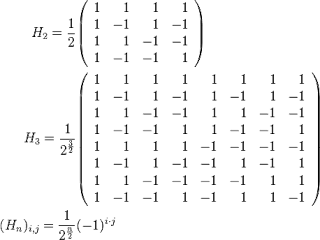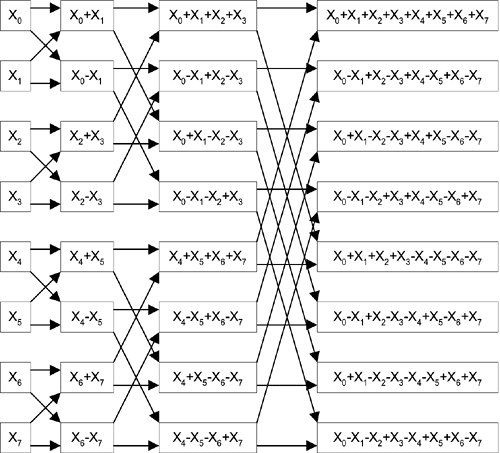## Artificial neural networks

An artificial neuron.
The basic behavior of an artificial neural network is determined by the dot product (weighted sum) operator in each neuron.
This also has a geometric interpretation.
The dot product of A and B is the magnitude (vector length) of vector A by the magnitude of vector B by the cosine of the angle between them.

Dot product video.

#### The dot product as linear associative memory

You can create a linear associative memory to provide scalar value responses to pattern vectors <pattern, response> by performing a dot product between the pattern vector and some adjustable weight vector.

If you use it to store 1 <pattern,response> item the angle between the pattern vector and the weight vector will be zero and the magnitude of the weight vector will adjust to give the necessary dot product response value.

If you use it to store 2 <pattern,response> items the angles between the pattern vectors and the weight vectors will both generally be non-zero.  Because the output of the dot product falls as angles increase toward 90 degrees the magnitude of the weight vector must be larger than is required to store either <pattern,response> on its own.

As more and more items are stored in the dot product the angle of the weight vector with respect to each of the pattern vectors needs to be repeatedly adjusted and its magnitude repeatedly increased.  At some stage there is no adjustment of the weight vector that can fit all the <pattern,response> items exactly.

Statistical properties of the dot product.

If a single <pattern,response> item is stored in a dot product and all the elements of the weight vector happen to be about equal in magnitude then there is quite a strong error correction effect, in fact in that case the central limit theorem applies.
The central limit theorem.
The noise in the output of the dot product is scaled down by a factor of about 1/sqr(n) for noisy pattern inputs, where n is the dimension of the dot product.

If 2 <pattern,response> items are stored the magnitude of the weight vector must increase.  This also multiples up the noise at the output of the dot product   to c/sqr(n) where c>1 for noisy inputs.  At some stage as more items are stored c=sqr(n) and the noise reduction effect ceases.

In general then for an under capacity linear associative memory you will get some noise reduction (error correction.)  At capacity you will get perfect recall but no noise reduction.  Over capacity you will get imperfect recall with fixed errors drawn from the Gaussian distribution.  The capacity for nicely distributed pattern vectors is around the dimension of the dot product, more skewed patterns may need pre-processing to get full capacity.
A final point is that any input pattern vector that is at a small angular distance to the weight vector is a super-normal stimuli that is likely to produce a super-normal response if more that 1 <pattern,response> item has been stored.

#### A more general associative memory

The dot product as a linear associative memory prefers patterns spread out over all the elements of the pattern vector for noise reduction (cf. The variance equation for linear combinations of random variables.)  There are also certain patterns that linear systems cannot distinguish between (linear separation issues.)
To deal with the first issue you can use some sort of vector to vector scrambling system prior to the dot product.
To deal with the second issue you can include nonlinear behavior prior to the dot product. A binary threshold function with bipolar (+1,-1) output is suitable and makes training the associative memory easy.
Eg.  https://github.com/S6Regen/Associative-Memory

#### Deep ReLU neural networks

The next component of an artificial neuron is the nonlinear activation function.
The most enlightening choice is the Rectified Linear Unit (ReLU.)ReLU activation function.The complete neuron.
An electrical switch is 1 volt in 1 volt out, n volts in n volts out when on, zero volts out when off.
An ReLU is a switch that is off when its input is less than zero, and a switch that is on when its input is greater than zero.  In a neuron it either gives zero out or the result of a dot product.ReLU is a literal switch.A ReLU neural network.
The dot product of a number of dot products is still a linear system.
For example given 3 dot products involving x,y and z:
u=a.x+b.y+c.z
v=d.x+e.y+f.z
w=g.x+h.y+i.z
A possible dot product of those is:
x=3.u+2.v+1.w
x=(3.a+2.d+1.g).x+(3.b+2.e+1.h).y+(3.c+2.f+1.i).z
Which is again just a simple dot product involving x,y and z.

A ReLU neural network then is a switched system of linear projections.  For a particular input each of the ReLU switches is decidedly on or off and there is a particular linear projection from the input vector to the output vector in effect.

For a particular input and a particular output neuron there is a particular combination of dot products reaching back to the input vector in effect.
Those dot products can be combined into a single effective dot product of the input vector.

For a particular input and a particular ReLU there is a particular combination of dot products fed to it on which it will make a switching decision.  And that combination can be condensed into into a single effective dot product of the input vector.  Looking at that condensed dot product you can gain some idea of what the ReLU is looking at in the input to make its decision.  In all probability it is going to look at some texture in some particular place in the input vector since that is the kind of thing dot products are good at.  Which explains the well known observation that artificial neural networks are rather sensitive to texture.

#### Efficient dot product algorithms

Since ReLU neural networks are just switched systems of dot products you may consider incorporating efficient dot product algorithms such as the FFT or the Walsh Hadamard transform (WHT) into such networks or in fact constructing networks based entirely on them.The 4-point (H2) and the 8-point (H3) WHT.
Multiplying a column vector by the 4-point Walsh Hadamard matrix gives 4 separate dot products and takes 4*4 (16) fused multiply adds.  The 8-point WHT (H3) takes 8*8 (64) fused multiply adds.
Using a fast WHT algorithm the operation count can be cut from n*n to n*log_base_2(n) operations.  Also multiplies by 1 or -1 convert to either nothing or simple negation.The fast 8-point in-place WHT algorithm.
The 4-point transform can be done in 4*2 operations, the 8-point in 8*3 operations.  The 65536-point transform in 65536*16 operations compared to 65536*65536 (4294967296) operations using the matrix form.

The n weighted sums in a conventional neural network layer of width n also require n*n fused multiply adds.  Which is rather costly.

The ReLU function is not parameterized, there is nothing adjustable about it. However if you allow such a thing then the individually adjustable switch slope at zero function f(x)=a.x  x>=0, f(x)=b.x  x<0 is quite similar to ReLU and also dot product compatible.
Combining that with the WHT then one possible neural network architecture is:

A condensed discussion (hint sheet) about the WHT is here:

Associative Memory (Press 1 to start and stop training.)

Further information (kinda the same):

#### Fin.

1.The simple WHT based neural networks with the parameterized switch slope at zero functions benefit from initialization with a constant, say 0.5 for all the parameters. That allows complexity to build up over time instead of trying to unscramble a massively complex mess right from the get go that happens with random initialization. At least that is so if you use evolutionary algorithms to train the system. I don't know what the situation would be with back-propagation. Basically constant initialization speeds up training using evolution by rather a lot.

2.You can help evolution based training algorithms even further by using a soft switching parameterized function such as f(x)=a*x x>1, f(x)=b*x x<-1, p=0.5*(x+1) q=1-p f(x)=x*(p*a+q*b) otherwise.

3.The associative memory can be used for Neural Turing Machines. That is neural networks with an external memory bank.

The WHT based neural network shows strong GAN like behavior when trained as an autoencoder and fed random inputs.